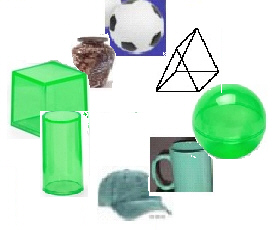KCC5.2
 Name:    KCC5.2

Multiple Choice
Identify the choice that best completes the statement or answers the question.

1.

Tell how many.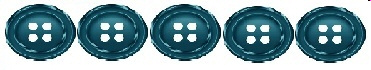a. 8 c. 6 b. 3 d. 5

2.

Tell how many.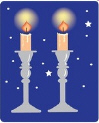a. 4 c. 2 b. 3 d. 1

3.

Which shows 11?
 a.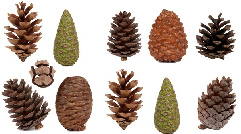c.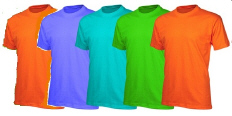b.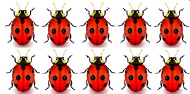d.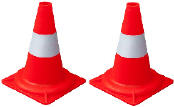4.

Tell how many.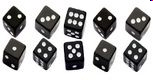a. 9 c. 12 b. 10 d. 7

5.

Which shows 6?
 a.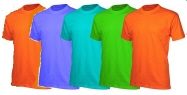c.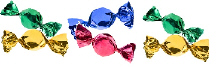b.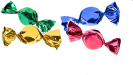d.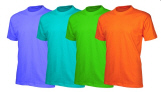Completion
Complete each statement.

6.

How many rectangles are there?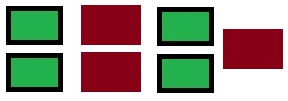7.

How many objects are there?